### Relation of ARMA and State Space Forms

Every state space model has an ARMA representation, and conversely every ARMA model has a state space representation. This section discusses this equivalence. The following material is adapted from Akaike (1974), where there is a more complete discussion. Pham-Dinh-Tuan (1978) also contains a discussion of this material.

Suppose you are given the following ARMA model: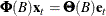or, in more detail,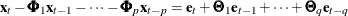(1)

where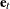is a sequence of independent multivariate normal random vectors with mean 0 and variance matrix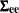, B is the backshift operator (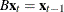),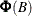and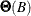are matrix polynomials in B, and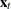is the observed process.

If the roots of the determinantial equation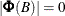are outside the unit circle in the complex plane, the model can also be written as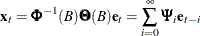The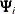matrices are known as the impulse response matrices and can be computed as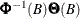.

You can assume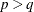since, if this is not initially true, you can add more terms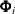that are identically 0 without changing the model.

To write this set of equations in a state space form, proceed as follows. Let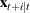be the conditional expectation of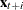given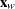for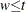. The following relations hold: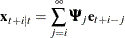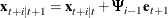However, from equation (1) you can derive the following relationship: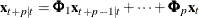(2)

Hence, when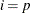, you can substitute for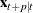in the right-hand side of equation (2) and close the system of equations.

This substitution results in the following model in the state space form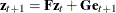: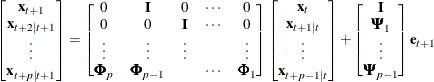Note that the state vector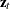is composed of conditional expectations ofand the first r components ofare equal to.

The state space form can be cast into an ARMA form by solving the system of difference equations for the first r components.

When converting from an ARMA form to a state space form, you can generate a state vector larger than needed; that is, the state space model might not be a minimal representation. When going from a state space form to an ARMA form, you can have nontrivial common factors in the autoregressive and moving average operators that yield an ARMA model larger than necessary.

If the state space form used is not a minimal representation, some but not all components ofmight be linearly dependent. This situation corresponds to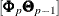being of less than full rank whenandhave no common nontrivial left factors. In this case,consists of a subset of the possible components of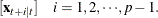However, once a component of(for example, the jth one) is linearly dependent on the previous conditional expectations, then all subsequent jth components of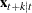for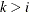must also be linearly dependent. Note that in this case, equivalent but seemingly different structures can arise if the order of the components withinis changed.